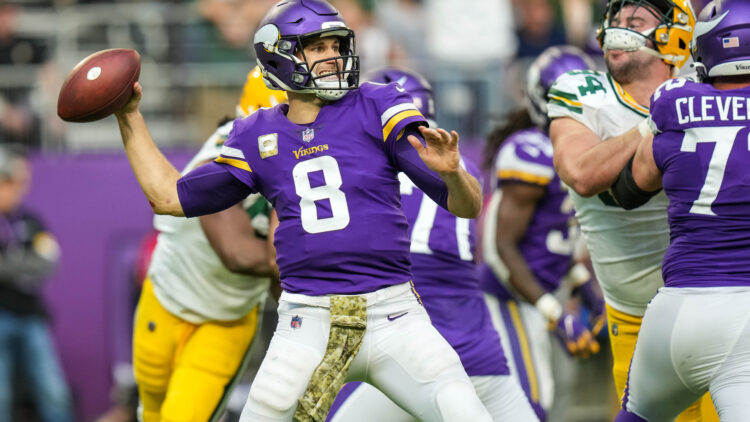# NFL’s Top QBs after Week 11: PFF, QBR, Passer Rating Formula#### The following scores are “good” through November 24, 2021..

There is a healthy fight within the stat community on which quarterback metric is most coherent. Passer rating is bashed as too antiquated. When used to compare quarterbacks from eras of yesteryear, passer rating is indeed skewed and inflated. But touchdowns, passing yards, interception, and completion percentage will never be wholly irrelevant.

ESPN’s QBR is said to factor in more intangible-type ratings. It can be interpreted as a more detailed and comprehensive version of passer rating and then some.

Pro Football Focus uses its grading system to assess the x’s and o’s of each game and pump out a yearly score.

The following scores and rankings are weighted. Passer rating accounts for 20% of the score, QBR at 40%, and PFF at 40%. Skill position players and players with menial pass attempts were excluded.

## 37. Zach Wilson (NYJ), 47.3

PFF = 59.5, QBR = 27.0, P-Rating = 63.5

## 36. Justin Fields (CHI), 49.8

PFF = 64.2, QBR = 25.8, P-Rating = 69.0

## 35. Jared Goff (DET), 51.3

PFF = 59.5, QBR = 26.8, P-Rating = 84.0

## 34. Davis Mills (HOU), 51.9

PFF = 59.3, QBR = 30.5, P-Rating = 80.2

## 33. Trevor Lawrence (JAX), 52.0

PFF = 58.8, QBR = 35.0, P-Rating = 72.8

## 32. Sam Darnold (CAR), 53.5

PFF = 62.6, QBR = 35.6, P-Rating = 71.3

## 31. Mike White (NYJ), 57.2

PFF = 53.6, QBR = 52.0, P-Rating = 75.1

## 30. Ben Roethlisberger (PIT), 59.1

PFF = 58.1, QBR = 43.8, P-Rating = 91.7

## 29. Trevor Siemian (NO), 59.9

PFF = 68.6, QBR = 36.9, P-Rating = 88.9

## 28. Baker Mayfield (CLE), 60.3

PFF = 68.1, QBR = 37.2, P-Rating = 91.3

## 27. Daniel Jones (NYG), 61.6

PFF = 71.4, QBR = 40.8, P-Rating = 84.0

## 26. Tyrod Taylor (HOU), 62.2

PFF = 62.2, QBR = 53.3, P-Rating = 80.3

## 25. Taylor Heinicke (WAS), 63.3

PFF = 64.1, QBR = 48.5, P-Rating = 91.4

## 24. Jacoby Brissett (MIA), 66.0

PFF = 76.0, QBR = 50.1, P-Rating = 78.1

## 23. Geno Smith (SEA), 66.6

PFF = 73.9, QBR = 41.3, P-Rating = 103.0

## 22. Russell Wilson (SEA), 68.9

PFF = 76.1, QBR = 45.6, P-Rating = 101.2

## 21. Carson Wentz (IND), 69.6

PFF = 69.5, QBR = 56.1, P-Rating = 97.2

## 20. Teddy Bridgewater (DEN), 69.9

PFF = 78.2, QBR = 47.3, P-Rating = 98.8

## 19. Patrick Mahomes (KC), 71.3

PFF = 73.7, QBR = 57.2, P-Rating = 94.7

## t18. Matt Ryan (ATL), 71.3

PFF = 81.1, QBR = 52.3, P-Rating = 89.8

## t18. Tua Tagovailoa (MIA), 71.3

PFF = 74.6, QBR = 58.0, P-Rating = 91.4

## 16. Lamar Jackson (BAL), 71.6

PFF = 78.1, QBR = 54.4, P-Rating = 93.1

## 15. Jalen Hurts (PHI), 71.7

PFF = 82.2, QBR = 52.0, P-Rating = 90.4

## 14. Ryan Tannehill (TEN), 72.0

PFF = 83.1, QBR = 54.0, P-Rating = 86.1

## 13. Jimmy Garoppolo (SF), 72.4

PFF = 71.6, QBR = 59.6, P-Rating = 100.0

## 12. Derek Carr (LV), 72.8

PFF = 79.9, QBR = 53.7, P-Rating = 96.8

## 11. Joe Burrow (CIN), 73.0

PFF = 82.5, QBR = 49.4, P-Rating = 101.6

## 10. Mac Jones (NE), 73.7

PFF = 86.9, QBR = 50.2, P-Rating = 94.7

## 9. Dak Prescott (DAL), 74.7

PFF = 83.7, QBR = 51.3, P-Rating = 103.9

## 8. Josh Allen (BUF), 75.1

PFF = 80.6, QBR = 58.7, P-Rating = 97.1

## 7. Jameis Winston (NO), 75.9

PFF = 74.2, QBR = 64.3, P-Rating = 102.8

## 6. Aaron Rodgers (GB), 79.1

PFF = 79.3, QBR = 65.3, P-Rating = 106.6

## 5. Matthew Stafford (LAR), 79.4

PFF = 77.7, QBR = 67.9, P-Rating = 106.1

## 4. Kirk Cousins (MIN), 80.9

PFF = 90.2, QBR = 59.1, P-Rating = 106.3

## 3. Kyler Murray (ARI), 81.6

PFF = 85.6, QBR = 63.3, P-Rating = 110.4

## 2. Justin Herbert (LAC), 82.0

PFF = 89.0, QBR = 67.0, P-Rating = 98.4

## 1. Tom Brady (TB), 83.7

PFF = 91.5, QBR = 65.8, P-Rating = 104.3

Dustin Baker is a political scientist who graduated from the University of Minnesota in 2007. He hosts a podcast with Bryant McKinnie, which airs every Wednesday with Raun Sawh and Sally from Minneapolis. His Viking fandom dates back to 1996. Listed guilty pleasures: Peanut Butter Ice Cream, ‘The Sopranos,’ and The Doors (the band).

Share: